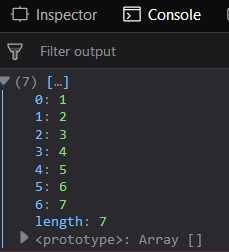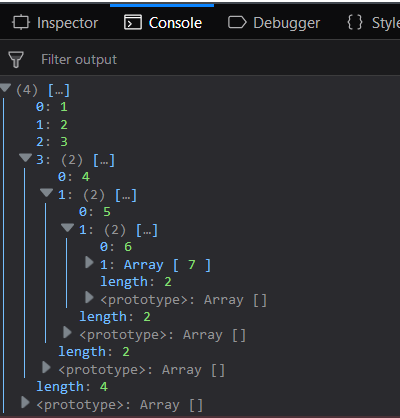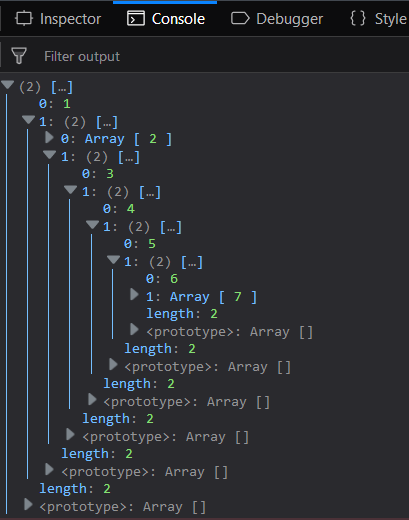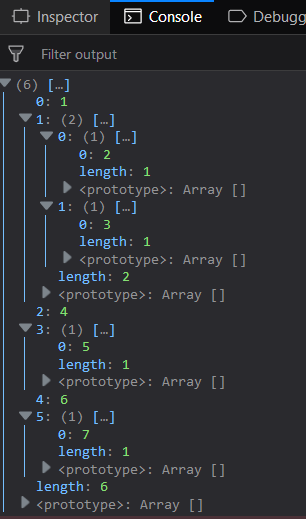# Underscore.js | _.flatten() with Examples

The _.flatten() function is an inbuilt function in Underscore.js library of JavaScript which is used to flatten an array which is nested to some level. The resultant array will have no depth. It will be completely flattened. If pass the shallow parameter then the flattening will be done only till one level. The depth of an array is given by the number of square brackets. Example: array[10, ] contains 10 element of 1 depth as it is inside only a single bracket ([]) whereas the element 20 has depth 2.

Syntax:

`_.flatten(array, [shallow])`

Parameters: This function contains two parameters which are listed below:

• array: This parameter is used to hold the array elements.
• shallow: It is used to flattened an array into single level.

Return value: It returns the flattened array either one level or all levels.

Passing a list without shallow parameter _.flatten() function: The _.flatten() function is used to make the nested array flattened. It will take the array and remove all its depth to make it at 1 level. Since, the second parameter is not given so the all it’s depth will be reduced.

Example:

 ` ` `<``html``> ` `    ``<``head``> ` `        ``<``script` `src` `=  ` `"https://cdnjs.cloudflare.com/ajax/libs/underscore.js/1.9.1/underscore-min.js"` `> ` `        `` ` `    `` ` `    ``<``body``> ` `        ``<``script` `type``=``"text/javascript"``> ` `            ``console.log(_.flatten([1, , [3, [4, [5, [6, ]]]]])); ` `        `` ` `    `` ` `                     `

Output:Passing the second parameter to the _.flatten() function: Passing the second parameter to the function so the n depth array will be flattened to n-1 depth. In the below example, the elements 1, 2 and 3 are enclosed inside only 1 bracket so they will no depth. The element 4 has 2 depths in the given array so it will have only 1 depth now and hence it will not be seen with the 1, 2 and 3 elements. the 5 element has 3 depth so in the resultant array will have 2 depth. Similarly, depth of 6th element is 6. And the last element 7 has 5 depth so it will be displayed at the inner most array.

Example:

 ` ` `<``html``> ` `    ``<``head``> ` `        ``<``script` `src` `=  ` `"https://cdnjs.cloudflare.com/ajax/libs/underscore.js/1.9.1/underscore-min.js"` `> ` `        `` ` `    `` ` `    ``<``body``> ` `        ``<``script` `type``=``"text/javascript"``> ` `            ``console.log(_.flatten([1, , [3, [4, [5, [6, ]]]]], true)); ` `        `` ` `    `` ` `                     `

Output:Another Example using shallow parameter: In this example element 1 has depth 1 so it will be displayed at the starting level along with an array of 2 elements. The 1st element contains 2 element, where as the second element again has 2 elements. The first element is the 3 and the second also is having 2 elements in an array. Likewise the array also has 4 and an array of elements. Keep going like this, then the final array will contain element 7.

Example:

 `<``html``> ` `  `  `<``head``> ` `    ``<``script` `src` `=  ` `"https://cdnjs.cloudflare.com/ajax/libs/underscore.js/1.9.1/underscore-min.js"` `> ` `    `` ` ` ` `  `  `<``body``> ` `    ``<``script` `type``=``"text/javascript"``> ` `        ``console.log(_.flatten([1, [[, [3, [4, [5, [6, ]]]]]]], true)); ` `    `` ` ` ` `  `  ` `

Output:Another example to the _.flatten() function with shallow parameter: In this example the elements 1, 4, 6 can be seen at the first level as their depth is 1. Other than these the first level contains an array of 2 elements and 2 arrays of 1 element each. The array of size 2 contains element 2 and 3 at the next level. One of the array of one size contains 5 and the other contains 7 at the next level as their original depth was 2.

Example:

 ` ` `<``html``> ` `    ``<``head``> ` `        ``<``script` `src` `=  ` `"https://cdnjs.cloudflare.com/ajax/libs/underscore.js/1.9.1/underscore-min.js"` `> ` `        `` ` `    `` ` `    ``<``body``> ` `        ``<``script` `type``=``"text/javascript"``> ` `            ``console.log(_.flatten([1, [[, ]], [4, ], [6, ]], true)); ` `        `` ` `    `` ` `                     `

Output:My Personal Notes arrow_drop_upCheck out this Author's contributed articles.

If you like GeeksforGeeks and would like to contribute, you can also write an article using contribute.geeksforgeeks.org or mail your article to contribute@geeksforgeeks.org. See your article appearing on the GeeksforGeeks main page and help other Geeks.

Please Improve this article if you find anything incorrect by clicking on the "Improve Article" button below.

Article Tags :

Be the First to upvote.

Please write to us at contribute@geeksforgeeks.org to report any issue with the above content.